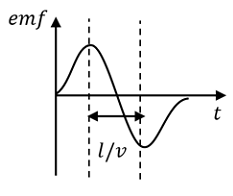Win up to 100% scholarship on Aakash BYJU'S JEE/NEET courses with ABNAT Win up to 100% scholarship on Aakash BYJU'S JEE/NEET courses with ABNAT

# JEE Main 2021 August 27th Shift 1 Physics Question Paper

JEE Main 2021 August 27 Shift 1 Physics Question Paper with Solutions is available here. The questions are based on Class XI and Class XII Physics syllabus. The question paper includes questions from topics like Current electricity, Capacitors, Sound, Thermodynamics, Electromagnetic waves etc. The answers provided here by the subject experts at BYJU’S are detailed and easy to understand. The JEE Main 2021 August 27 Shift 1 Physics Question Paper with Solutions are available in PDF format for candidates to download for offline reference.

JEE Main 2021 August 27th Shift 1 Physics Question Paper

Question 1: A conducting wire of length 24a is used to form two setups of coils, first in the form of a square of side a and second in the form of an equilateral triangle of side a. Find the ratio of the magnetic moment of the coil in two cases?

a. √3/1
b. √2/1
c.

$$\begin{array}{l}\sqrt{\frac{3}{5}}\end{array}$$

d.
$$\begin{array}{l}\sqrt{\frac{5}{3}}\end{array}$$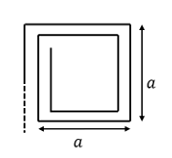4anr = 24a

Thus, n1 = 6 turns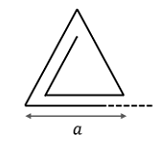3anz = 24 ⇒ nz = 8 turns

$$\begin{array}{l}\frac{M_{1}}{M_{2}}=\frac{n_{1}iA_{1}}{n_{2}iA_{2}}=\frac{6 \times 1^{2}}{8\times a^{2}\times \frac{\sqrt{3}}{4}}= \frac{\sqrt{3}}{1}\end{array}$$

Question 2: A particle of mass 2 M is divided into four particles of mass m, m, M – m and M – m. All particle is arranged on the vertex of the square of side a. Find the ratio of M/m, for which potential energy of this system is;

a. 2
b. 1/2
c. 3
d. ⅓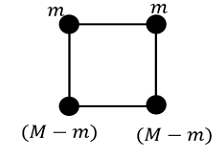$$\begin{array}{l}u=\frac{Gm^{2}}{a}+\frac{G(M-m)^{2}}{a}+\frac{2Gm(M-m0)}{a}+\frac{2G(M-m)}{\sqrt{2}a}\end{array}$$

For maxima or minima of potential energy (dU/dm)= 0

dU/dm = (G/a)(2m − 2(M − m) + (M − 2m)2 + √2 (M − 2m)

(4m − 4m − 2 √2m) + (−2M + 2M + √2 M) = 0; √2 M = 2 √2 m;M/m = 2

Question 3: A source A produce frequency of horn 1120 Hz. Source and observer are moving towards each other with speeds of 36 km/hr and 72 km/hr respectively. Find frequency of horn observed by the observer (speed of sound V = 330 m/s).

a. 1210 Hz
b. 1200 Hz
c. 1225 Hz
d. 1250 Hz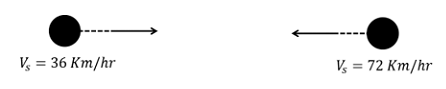Vs = 36 km/hr

= 10 m/s

V0 = 72 km/hr

= 20 m/s

f′ = f0 {(V+V0)/(V−VS)}

f′ = 1120 [(330+21)/(330−10)] = 1120 ×(35/32)

= 1225 Hz

Question 4: For an ideal gas PT3 = constant. Find the coefficient of volume expansion:

a.1/T
b. 2/T
c. 3/T
d. 4/T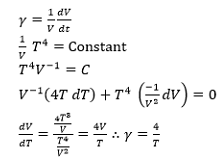Question 5: Find q on 4 μf capacity at t = ∞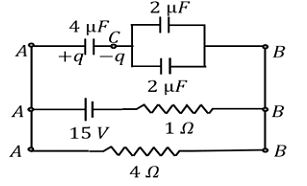a. 8 μC
b. 12 μC
c. 20 μC
d. 24 μC

Using i = 15/(4+1) = 3 A

Therefore, VAB = i × 4 = 12 V

VAC = VCB = 6 Volt

Therefore, q on 4 μF = CVAC = 24 μC

Question 6: n identical resistance each having value 10 Ω are connected in series with a battery of emf 20 V & internal resistance 10 Ω. The current in a circuit is is. If they are connected in parallel to the same source current is ip. If ip = 20 − is then n will be:

a. 10
b. 8
c. 12
d. 20

Given,

ip = 20 is

$$\begin{array}{l}\Rightarrow \frac{20}{\frac{10}{n}+10}= 20(\frac{20}{10n+10})\end{array}$$
$$\begin{array}{l}\Rightarrow \frac{20}{10+10n}= 20(\frac{20}{10n+10})\end{array}$$

Therefore, n = 20

Question 7: The height of the transmission and receiver tower are 380 m and 800 m respectively. If the radius of the earth is 6400 km, then find the range of LOS communication.

a. 150 km
b. 171 km
c. 250 km
d. 200 km

$$\begin{array}{l}Range = \sqrt{2Rh_{1}}+\sqrt{2Rh_{2}}=\sqrt{2\times 6400\times 380}+\sqrt{2\times 6400\times 800}\approx 171 km\end{array}$$

Question 8: An electromagnetic wave is given by E = sin(50 x + 1010t)V/m. Find speed in terms of C.

a. 2/3C
b. 1/2C
c. ¼ C
d. ¾ C

We can conclude from the given equation

ω = 1010

k = 50

Speed = ω/k =1010/50

= 2 × 108 =⅔ C

Question 9: If E is electric field intensity and H is the magnetic intensity then find the SI unit of E/H:

a. Joule (second – Ampere2)
b. Ampere/(second2)
c. Joule /Ampere2
d. Ampere/meter

$$\begin{array}{l}\frac{E}{H}=\frac{\frac{F}{q}}{\frac{1}{q}}=\frac{Fr}{I^{2}t}\end{array}$$

= Joule (second – Ampere2)

Question 10: When F1 force is applied at an angle 450 w.r.t direction of displacement, work done by the force, in this case, is equal to work done when a force F2 is applied on the same block at an angle 600 w.r.t direction of displacement. If displacement in both the cases is the same, the ratio of F1/F2 is 1/√x, find x?

Since displacement in both the cases is the same, let it be s

Also, w1 = w2

⇒ F1s cos 450= F2s cos 600

⇒F1/F2 =1/√2

⇒ x = 2

Question 11: A disc having uniform surface charge density σ is placed in y – z with centre at origin and radius R. Find the electric field at a point (x,0,0).

a.

$$\begin{array}{l}E_{x}=\frac{\sigma }{2\varepsilon _{0}}\left [ 1-\frac{x}{\sqrt{R^{2}+x^{2}}} \right ]\end{array}$$

b.
$$\begin{array}{l}E_{x}=\frac{\sigma }{\varepsilon _{0}}\left [ 1-\frac{\sqrt{R^{2}+x^{2}}}{x} \right ]\end{array}$$

c.
$$\begin{array}{l}E_{x}=\frac{\sigma }{\varepsilon _{0}}\left [ 1-\frac{x}{\sqrt{R^{2}+x^{2}}} \right ]\end{array}$$

d.
$$\begin{array}{l}E_{x}=\frac{\sigma }{2\varepsilon _{0}}\left [ x-\frac{x}{\sqrt{R^{2}+x^{2}}} \right ]\end{array}$$

The disc can be considered to be a collection of a large number of concentric rings.

Consider an element of the shape of rings of radius r and of width dr. Electric field due

to this ring at P is;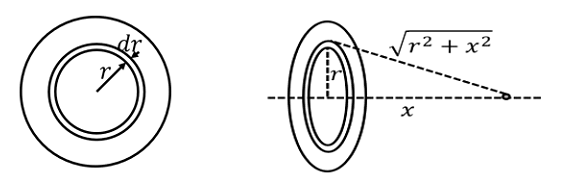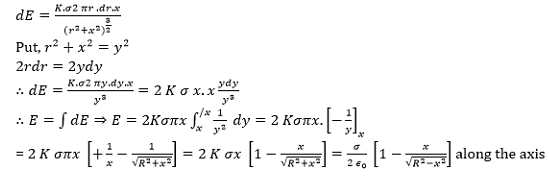Question 12: Find the radius of curvature of a concave mirror. If the object is at d1 distance away from Pole and image formed is at d2 distance away from Pole: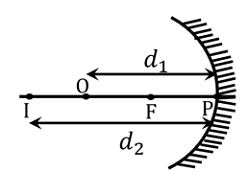a. (2d1d2)/(d1+d2)
b. d1d2/(d1+d2)
c. d1d2/(2d1+d2)
d. d1d2/(d1+2d2)

Using Newton’s formula, we have

xy = f2

(d1 − f)(d2 − f) = f2

f = d1d2/(d1+d2)

Radius of curvature, Roc = 2f =2d1d2/(d1+d2)

Question 13: Find the moment of inertia of a uniform square plate of mass M and side length ‘l’ about an axis passing through one of its vertices and perpendicular to plane?

a. Ml2/3
b. 2Ml2/3
c.Ml2/6
d. Ml2/12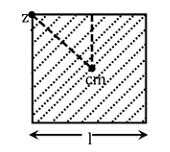$$\begin{array}{l}I_{z}=I_{OM}+M(\frac{l}{\sqrt{2}})^{2}\end{array}$$
$$\begin{array}{l}= \frac{Ml^{2}}{6}+\frac{Ml^{2}}{2}=\frac{4Ml^{2}}{6}=\frac{2Ml^{2}}{3}\end{array}$$

Question 14: Find the value of the resultant force shown in the figure.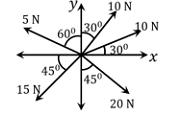a. −10.75 î− 8 ĵ
b. −10.75 î− 9 ĵ
c. −12.75 î− 7 ĵ
d. −15.75 î− 9 ĵ

Resultant = î(10 cos 300 + 10 cos 600 − 5 cos 300 − 15 cos 450 + 20 cos 450

+ ĵ(10 sin 300 + 10 sin 600 + 5 sin 300 − 15 sin 450 − 20 cos 450

= −15.75 î− 9 ĵ

Question 15: 5 same cells (5V, 1 Ω) connected in series and then in parallel with an external resistance R respectively if current in both circuits is equal then find the value of R.

a. 4 Ω
b. 3 Ω
c. 2 Ω
d. 1 Ω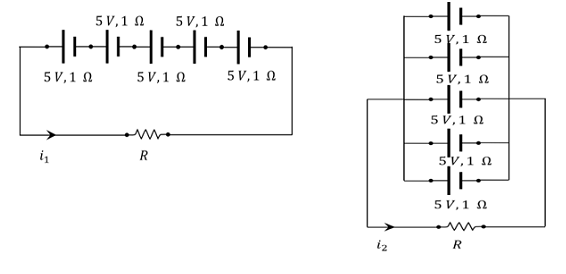$$\begin{array}{l}i_{1}=\frac{5\epsilon }{R+5r}i_{2}=\frac{\epsilon }{R+\frac{r}{5}}\end{array}$$

ir=iz

$$\begin{array}{l}\frac{5c}{R+5r}=\frac{\epsilon }{R+\frac{r}{5}}\end{array}$$

⇒ R = r = 1Ω

Question 16: A huge circular arc of length 4.4 ly subtends an angle ‘4S’ at the centre of the circle. How long it would take for a body to completed 4 revolutions if its speed is 8 Au per second?

a. 3.5 × 106 S
b. 4.5 × 106 S
c. 7.2 × 108 S
d. 4.1 × 108 S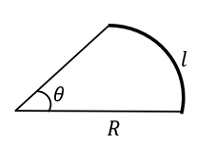l = 4.4 ly = 4.4 × 9.46 × 1015

Length of Arc = l = R θ

4.4 × 9.46 × 1015 = R θ

θ = 4S = 4 × 4.843 × 10−6 = 1.94 × 10−5 rad

4.4 × 9.46 × 1015 = R × 1.94 × 10−5

4 revolution means distance = 4 × 2 πR metere

Time =distance/speed

= (4×2 πR) /12×1011

time =(8×3.14×2.1455×1021)/(12×1011)

⇒ 4.5 × 1010 sec

Question 17:For the given arrangement, what will be the distance of the final image from the third lens?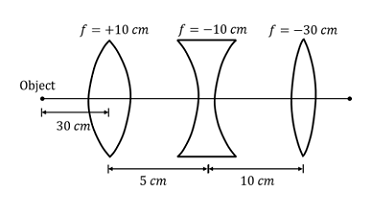a. 75
b. 30
c. ∞
d. 40

Lens formula

$$\begin{array}{l}\frac{1}{v}-\frac{1}{u}=\frac{1}{f}\end{array}$$
$$\begin{array}{l}\frac{1}{v}-\frac{1}{-30}=\frac{1}{10}\end{array}$$
$$\begin{array}{l}\frac{1}{v}=\frac{1}{10}-\frac{1}{30}\Rightarrow \frac{1}{v}=\frac{3-1}{30}\end{array}$$

v = 15 cm

Now we have u = + 10 cm

$$\begin{array}{l}\frac{1}{v}-\frac{1}{10}=\frac{1}{-10}\Rightarrow \frac{1}{v}= -\frac{1}{10}+\frac{1}{10}\end{array}$$

⇒v = ∞

It means for the third lens, rays are coming parallel to the principal axis, hence, an image will be formed at the focus of the third lens

Thus, V = + 30 cm (from third lens)

Question 18: Common Emitter transistor is used in which region as an amplifier.

a. Active region
b. Saturation region
c. Cut off region
d. Cut and saturation

Question 19: Initial number of active in a radioactive element is 1010. If the half-life of a radioactive element is 1 min. What will be the number of active nuclei after 30 sec? [e−0.25 = 0.70].

a. 0.7 × 108
b. 0.7 × 1010
c. 0.7 × 106
d. 0.7 × 109

$$\begin{array}{l}N =N_{o}e^{\frac{ln2.1}{\frac{r_{1}}{2}}}\end{array}$$

= 1010 e−0.35 = 0.75 × 1010

Question 20: For the given displacement – time curve of SHM. The potential – energy vs time curve will be?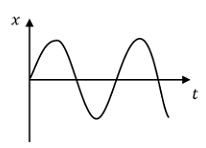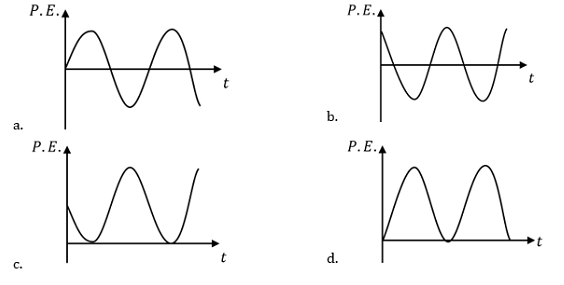Considering Spring – mass system

x = x0 sin ω t

P. E =(½)kx2 =1/2kx02sin2 ωt = c sin2 ωt

⇒ PE = (1−cos 2 ωt)/2

Thus, the value of P.E will always be positive, hence, as per the option graph in (d) is

correct.

Question 21: Two identical rods are arranged as shown in the figure. D is the midpoint of rod BC. Find the heat transfer rate in W through rod AD. (given,L/KA = 10 kw−1).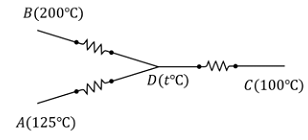ka(200−t)/(l/2) +KA (25−t)/l = [(t−100)KA]/(l/2)

400 − 2t + 125 − t = 2t − 200

725 = 5t

t = 1450C

$$\begin{array}{l}Q_{AD}=\frac{KA(125-145)}{l}=\left | \frac{-\frac{20}{L}}{\frac{l}{KA}}\right |=\left | \frac{20}{10}W \right |=2 W\end{array}$$

Question 22: A balloon carries a total load of 185 kg at normal pressure and temperature of 270C. What load with the balloon carry on rising to a height at which the barometer pressure is 45 cm of Hg and the temperature.

a. 123.54 kg
b. 219.07 kg
c. 214.15 kg
d. 181.46 kg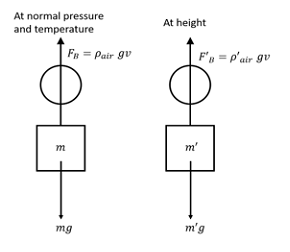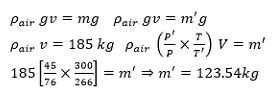Question 23: In a photoelectric effect, if the intensity of light is increased then;

a. Frequency increases
b. Number of photons increases
c. Kinetic energy of photoelectron increases
d. Momentum of photon increases

N = IA/hv

Hence, on an increase in the intensity of light, the number of photons will increase.

Question 24: In a circuit, current is given as i = √42 sin (4t/T) + 10 . Find rms current in circuit?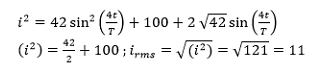Question 25: If velocity of a particle is given by v = √5000 + 24 x . Where x is displacement. The acceleration of particle is:

a. 12 m/s2
b. 15 m/s2
c. 18 m/s2
d. 21 m/s2

a =Vdv/dx

$$\begin{array}{l}v=\sqrt{5000+24x}\times \frac{1}{2\sqrt{5000}+24x}\times 24\end{array}$$

a = 12 m/s2

Question 26: A Bar magnet moving with velocity ‘V’ towards a fixed conducting circular loop. Find the graph of emf vs t. consider anticlockwise emf as positive emf where the observer is right of the south pole of the magnet.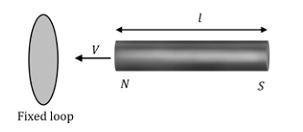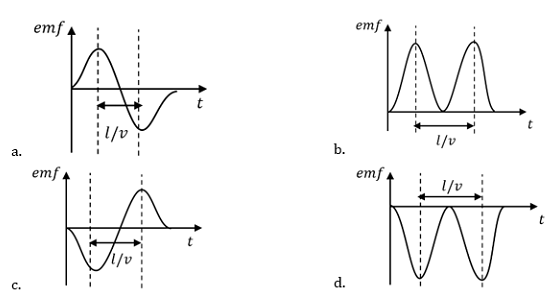As the magnet is approaching the loop, magnetic flux increases and the rate of increment of flux also increases so emf increases with time.

At this moment emf is maximum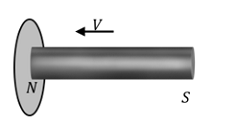Magnet is in the middle of the coil, at this moment emf is equal to zero.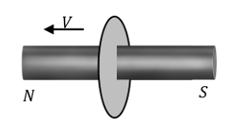Now, again flux start changing however polarity of emf get reverse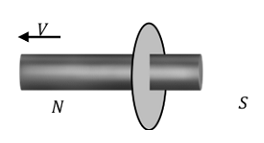At this moment emf is again maximum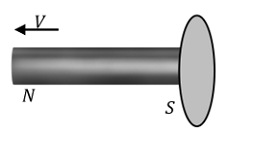Emf again becomes zero.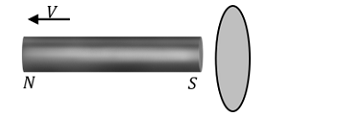Hence, the graph will be